# Intuitive and practical guide for building neural networks from scratch

#### Using perceptrons to solve university acceptance problem

This is the first blog of the article series as part of the community initiatives for AI India 2018 conference. The goal of this article series is to help the community to get an intuitive and practical insight into AI and build momentum leading up to the conference. The best part is that there is still 7 more months to go for the conference and its a great time to begin your AI journey.

Have you been curious about all the hype around AI but just couldn’t get started? Have you been making honest efforts to wrap your head around the math and basic concepts of AI but still find yourself at sea? Or you are just curious to get a practical, code based insight into fundamentals of AI and have some fun in the process? If so you are in the right place, let’s begin.

Here is the list of concepts which are covered in this blog,

• Perceptrons.
• Modelling the binary classification task using perceptron.
• Building AND gate using perceptrons.

Let’s get onto the problem statement right away.

#### The problem statement

We’ve got a dataset containing the university admission data. Here is how the data looks like, here is the link to the jupyter notebook cell. Just make a copy of the notebook to run it yourself on cloud.

The dataset contains 3 columns, the test scores, grades and the confirmation whether the student is accepted into the university or not. 1 represents the student being accepted and 0 being rejected.

We will solve the classification problem where the test scores and grades are the inputs and the output will be the prediction whether the student is accepted or not. Since the output is either of the 2 values, either accepted(represented as 1) or rejected (represented as 0), the problem technically can be termed as a binary classification problem.

Lets visualize the data, the ones in green represent the students who are accepted and the red ones are the those who are rejected. Here is the link to the notebook cell.

Lets start with a simple classification problem. We need a way to separate the red points from the green ones. The nature of the data from the plot shows us that the these points can be almost separated just by using a straight line. So here is the problem definition. We need to find out this straight which best separates the students who are accepted (in green), from those who are rejected ( in red). This line would also help us make future decision on whether to accept a student or not based on their test score and grades. Once the answer is found out it would look something like this,

We assume that there’s a functional mapping between the set of inputs and outputs, and during training we try to make sense of it and try to find that relationship.

Let’s learn some fundamentals which are necessary to solve the problem and then get back to crack it.

### Perceptrons

Perceptrons are the building blocks of neural networks, they are the basic units from which more complex neural networks are built. They can be used as simple linear classifiers, which can be used to draw a straight line to separate the linearly separable data points and that’s precisely what we want.

The perceptron is a mathematical model of a biological neuron.

Perceptron and neural networks?! It smells like some biological inspiration, isn’t it?. Yes, you are right and here’s why,

• While in actual neurons the dendrite receives electrical signals from the axons of other neurons, in the perceptron these electrical signals are represented as numerical values.
• At the synapses between the dendrite and axons, electrical signals are modulated in various amounts. This is also modeled in the perceptron by multiplying each input value by a value called the weight.
• An actual neuron fires an output signal only when the total strength of the input signals exceed a certain threshold. We model this phenomenon in a perceptron by calculating the weighted sum of the inputs to represent the total strength of the input signals, and applying a step function on the sum to determine its output.
• As in biological neural networks, this output is fed to other perceptrons.

The output of the perceptron is linear equation of inputs and weights. The important fact to notice is that its a linear equation.

• The values x1, x2… xn are the inputs to the perceptrons, these are represented as nodes of the perceptron network.
• The values w1, w2, …wn on the edges are called the weights of the network. These weights are like the knobs which controls the output value of the network.
• The weight w1 is associated with input x1, w2 with x2 and so on…
• The last node doesn’t correspond to any input features, it has a constant value of 1, the edge contains a value b called the bias of the network. Don’t worry much about the importance of bias, we’ll have better understanding about weights and bias as we progress further. For now consider it as a number without giving much heed to its importance.

The number of weights is equal to number of input features. And the output of the perceptron is either 0 or 1.

• The output of the network is calculated by first computing the product of the inputs and their corresponding weights and arriving at a score. And later run the score through a step function.
`score = w1 * x1 + w2 * x2 +  ..... + wn * xn + 1 * b`
`the output is `
`1  if the score > 00  if the score < 0`

Hold on for a moment !!!! How does perceptron help us find the straight line which help us separate the accepted and rejected candidates in case of the university admission problem?

That’s a valid question! Let’s figure that out so that it makes sense to continue to learn about perceptrons in order to crack our problem.

#### Modelling the problem using perceptrons

In the university admission problem which we are set out to solve there are 2 inputs, the test scores (x1) and grades (x2), we saw that the perceptron score is a linear function of inputs and weights, let w1 and w2 be the weights that for inputs x1 and x2, let a real number b be the bias of the network. Don’t forget that we already have ground truth label y, which represents whether the students with test score( x1) and grades (x2) are accepted or not.

Now according to the perceptron model the output of the network with inputs x1 and x2, and with weights w1 and w2 is

`score = w1 * x1 + w2 * x2 + b `
`Output is:      1 if score > 0     0 if score < 0`

In case of our university admission data an output of 1 corresponds to the student being accepted and 0 being rejected.

The goal is to learn the values w1, w2 and b in such a way that the perceptron network output (which is either 0 or 1) matches with the ground truth label y for most the training examples. We have 100 student records at our disposal for solving the university admission problem, we at-least expect that the output of perceptron matches with ground truth label for 90+ cases.

Once the values of w1, w2 and b are learned in a way which satisfies the goal the following equation represents the line which can best separate 2 classes of data.

`w1 * x1 + w2 * x2 + b = 0`

This fits the general equation of straight line which is represented by

`A * x + B * y + C = 0`

Check this link to know more about equations of a straight line.

Let’s consider an example, The following equation represents the straight line which separates the 2 classes of data, the accepted ones from the rejected ones for the university admission problem,

2×1 + x2–18 = 0 , where w1 = 2, w2 = 1 and c = -18.

Now you know the importance of having right set of values for w1, w2 and b. Because these parameters characterize the straight line.

Hence, finding the right set of parameters for the perceptron network which could best classify our dataset inturn gives us a straight decision boundary which best separates the two classes of data.

Still finding it hard to get the intuition? Well, don’t worry, let’s solve very simple problem using perceptrons and in the process let’s gain more intuition about how perceptrons work and how it can be used to draw a straight line boundary to separate 2 classes of data.

Let’s start with the simpler task of classifying the outputs of a 2 input AND gate using perceptrons,

Here’s how the input-output relationship looks like for an AND gate with 2 inputs. As you see the inputs are discrete and they are either 0 or 1. We need to find out the line which separates the input (1,1) with output 1 from rest of the inputs whose output is 0.

Let’s analyse the above training data first,

`1. We have 4 training examples.`
`2. Each training example has 2 input features, input_1 and input2.`
`3. Each training example has just 1 output.`
`4. The output is either 0 or 1, so it's a binary classification task.`

Let’s plot these 4 training examples and see how it looks, here is the link of the notebook cell, again, make a copy and run it yourself.

As you can see, the plot (1, 1) is in green, this corresponds to output value 1, and the other 3 input plots are in red indicating that their output is 0.

Here’s how we would to model the linear classification task for the AND gate dataset using the perceptron model,

`1. Choose a number for weights w1, w2 and bias b in a way that it satisfies the conditions mentioned in the points to follow. 2. The output of the perceptron      is 1 if w1 * input_1 + w2 * input_2 + b > 0     is 0 if w1 * input_1 + w2 * input_2 + b < 0`
`3. For the AND gate dataset we know the perceptron should output value of 1 only in case where both the inputs are 1. `
`4. Which means for inputs input_1 = 1 and input_2 = 1, the output of the perceptron w1 * input_1 + w2 * input_2 + b should be > 0, and for rest of the inputs the output of the perceptron should be < 0.`

The image below depicts the kind of straight line we are expecting for classifying the AND gate output, a line which can separate the input (1,1) from the rest.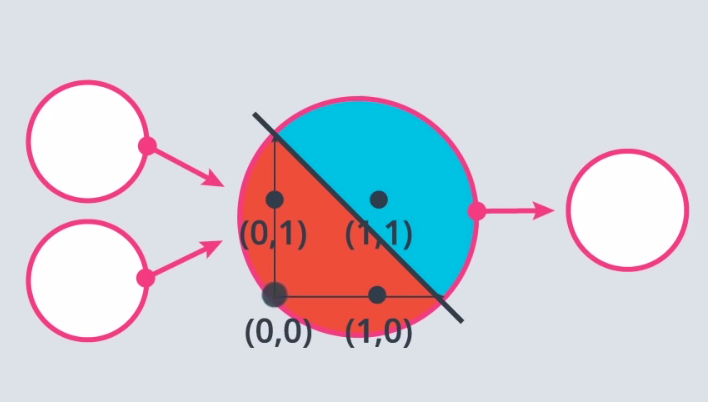This is the perceptron output we need for an AND gate, a straight line which separates input (1,1) from rest.

Refresher: That’s what a linear classifier for a binary classification task does, it separates the inputs whose outputs are 1 from those whose outputs are 0 by drawing a decision boundary using a straight line.

Let’s start with random values for w1, w2 and b to begin with and see how well it separates the 2 classes of data.

Set w1 = 3, w2 = 2, b = -1 to begin with, lets replace these values in the perceptron model and try couple of things,

1. First, check if the line represented by these parameters correctly separates data.
`# the decision boundary is represented by w1 * x1 + w2 * x2 + b = 0`
`# Replace w1, w2 and b with the random values we chose.# Here is our straight line equation.3 * x1 + 2 * x2 - 1 = 0`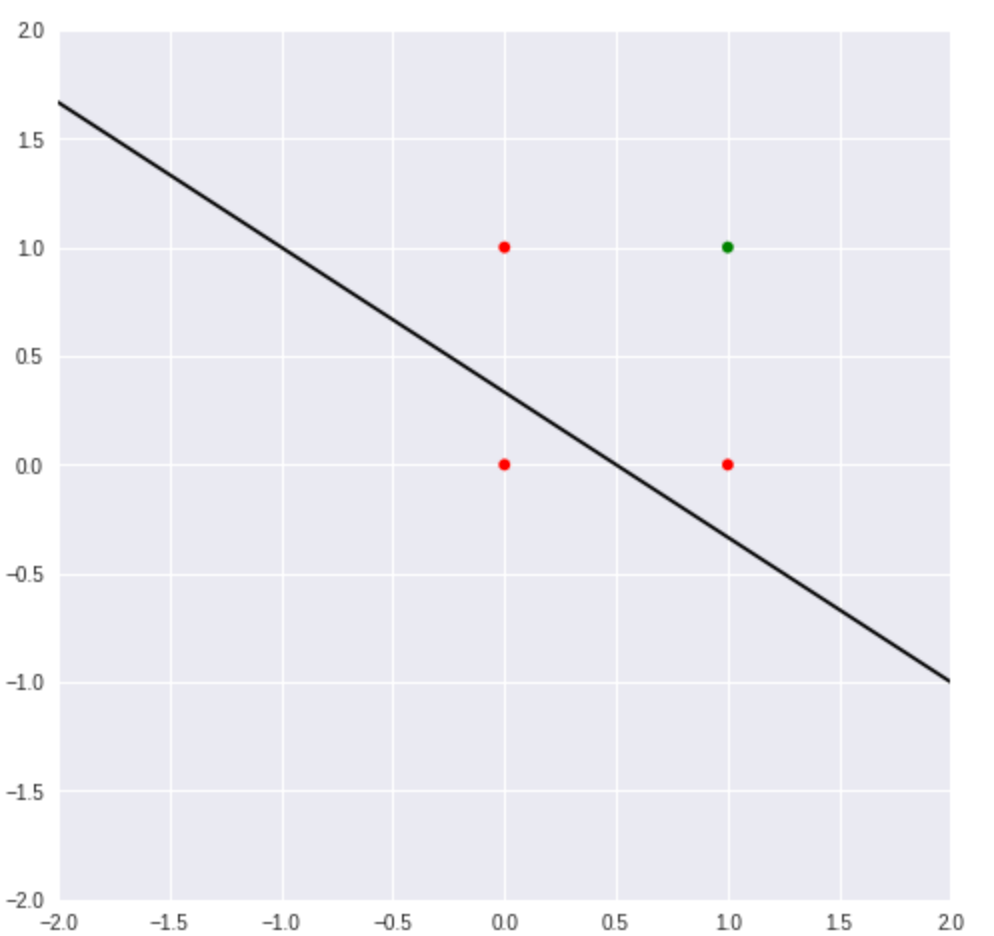The AND gate dataset and the straight represented by 3×1 + 2×2–1=0

Clearly the straight line 3 * x1 + 2 * x2 – 1=0 which is represented by parameters w1 = 3, w2 = 2 and b = -1 is not able to separate the red ones from green point.

Here is the link to the notebook cell, just make a copy and run on your own. Try the code with different values for w1, w2 and b.

Let’s check the perceptrons output for these parameters and compare it with the ground truth label.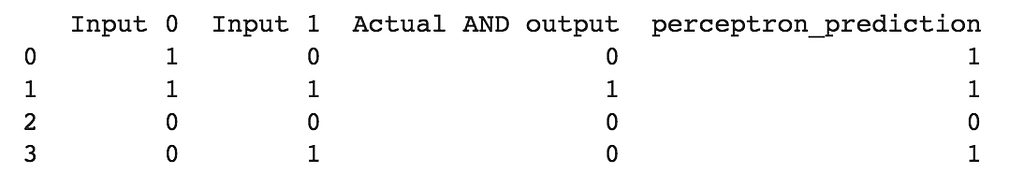The prediction output of perceptron for AND gate inputs, the parameters were w1 = 3, w2 = 2 and b = -1.

As you can clearly see in the table above the actual AND gate output and the perceptron prediction doesn’t match. Here is the link to notebook cell, make a copy of it and run it on your own, try various values of w1, w2 and b.

With parameters set to values w1 = 1, w2 = 1 and b = -1.5 the perceptron model is able to separate the 2 classes of data and predict the output correctly too. Here is the link to notebook cell,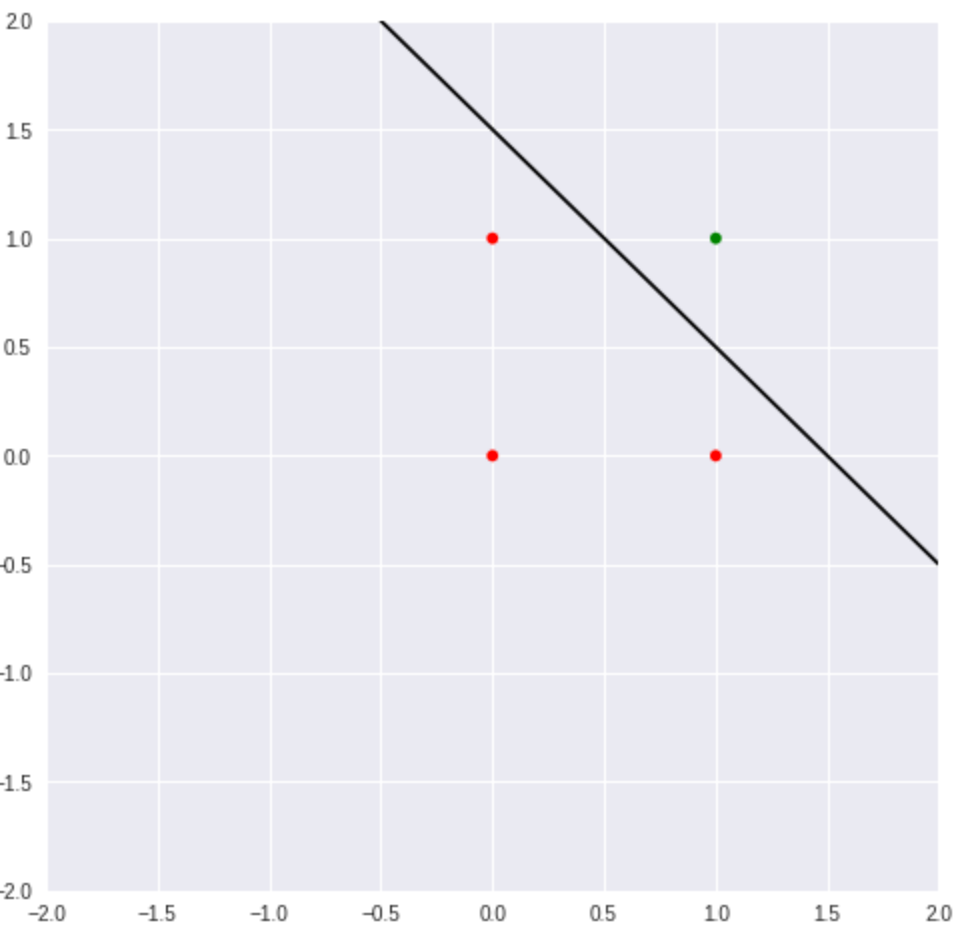With parameters w1 = 1, w2 = 1 and b = -1.5 the perceptron is able to separate the AND gate outputs

With parameters set to values w1 = 1, w2 = 1 and b = -1.5 the perceptron prediction matches the actual AND gate output, Here is the link to the notebook cell,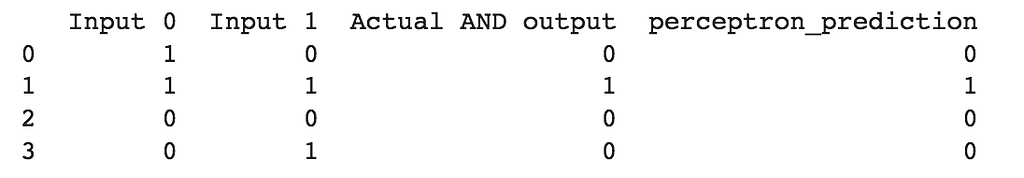The perceptron output matches the AND gate when parameters are set to values w1 = 1, w2 = 1 and b = -1.5

As an exercise try to set the parameters w1, w2 and b such that it satisfies the OR gate output.

But what about the initial problem we set out to solve? To draw the straight line to correctly classify or separate the accepted and rejected students?

In case of classifying AND gate output we set the parameters w1, w2 and b manually by hand. This brute force approach doesn’t scale when we have hundreds, thousands or sometimes millions of data points. And here we had only 2 inputs, how would we do it in case there’s a tens or even hundreds of output?

In the next post of the series we’ll learn about an algorithm which would learn the parameters (weights and bias) of the perceptron network on their own. We’ll have some fun learning some fundamental calculus too and then solve the university admission classification problem in an automated way.

Note: A lot of emphasis has been laid on making this blog really easy to be followed even for an absolute beginner. If there are any feedback on improving the blog please feel free to comment. If you find this useful don’t forget to clap and share. See you soon in the next blog. Till then, happy learning!